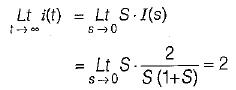Courses

# Laplace Transform - 1

## 10 Questions MCQ Test Topicwise Question Bank for GATE Electrical Engineering | Laplace Transform - 1

Description
This mock test of Laplace Transform - 1 for Electronics and Communication Engineering (ECE) helps you for every Electronics and Communication Engineering (ECE) entrance exam. This contains 10 Multiple Choice Questions for Electronics and Communication Engineering (ECE) Laplace Transform - 1 (mcq) to study with solutions a complete question bank. The solved questions answers in this Laplace Transform - 1 quiz give you a good mix of easy questions and tough questions. Electronics and Communication Engineering (ECE) students definitely take this Laplace Transform - 1 exercise for a better result in the exam. You can find other Laplace Transform - 1 extra questions, long questions & short questions for Electronics and Communication Engineering (ECE) on EduRev as well by searching above.
QUESTION: 1

Solution:
QUESTION: 2

Solution:
QUESTION: 3

### Which of the following is the correct Laplace transform of the signal in the given figure?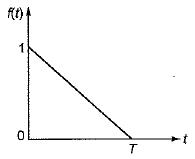Solution:
QUESTION: 4

Given: x(t) =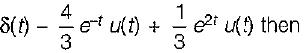find x(s) and its ROC:

Solution:
QUESTION: 5

Given: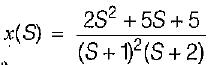and Re [S] >-1 then x (t):

Solution:
QUESTION: 6

If the input to LTI system is x(t) = e-3t u(t). Then the output is y(t) = [e-t - e-2t] u(t) the transfer function of the system is

Solution: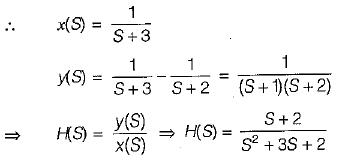QUESTION: 7

If,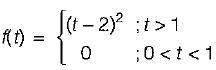then the value of L{tf(t)} is equal to

Solution:
QUESTION: 8

The transfer functions of the sytem is given by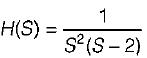The impulse response of the system is

Solution: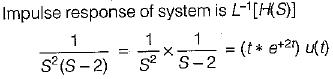QUESTION: 9

The Laplace transform of a continuous signal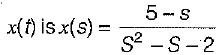If the Fourier transform of the signal exists, then x(t) is

Solution: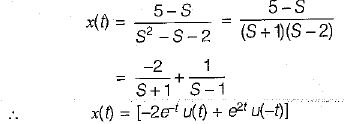QUESTION: 10

The laplace transform of i(t) is given by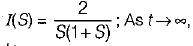the value of i(t) tends to

Solution: